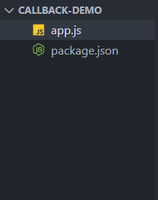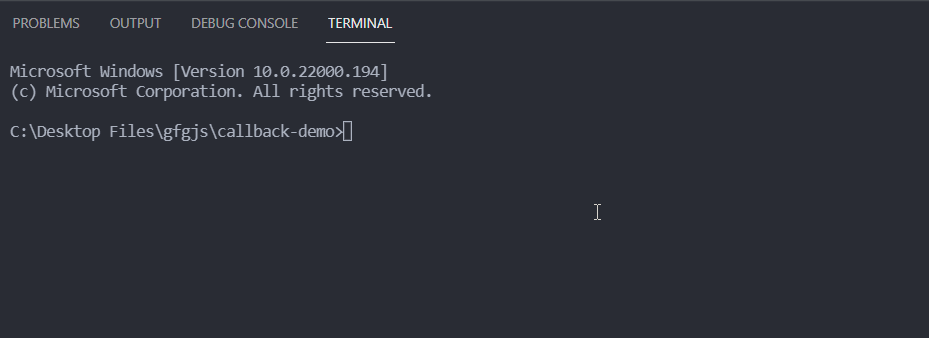# Which is first argument typically passed to a Node.js callback handler ?

• Last Updated : 10 Oct, 2021

A callback handler function in Node.js is a way to handle something after a particular operation is completed. It is one of the ways to handle asynchronous code which takes a long time to yield the result so we can call the callback handler with the error if any, and the result of the asynchronous operation.

The callback handler functions follow the error-first convention that is:

• The first argument of the callback handler should be the error and the second argument can be the result of the operation.
• While calling the callback function if there is an error we can call it like callback(err) otherwise we can call it like callback(null, result).

Syntax:

```const func = (arg1, agr2, ..., argN, callback) => {
// code logic
}

func(a, b, ..., n, (err, result) => {
// code logic
})```

Project Setup

Step 1: Install Node.js if you haven’t already.

Step 2:  Create a folder for your project and cd (change directory) into it. Create a new file named app.js inside that folder. Now, initialize a new Node.js project with default configurations using the following command.

`npm init -y`

Project Structure: After following the steps your project structure will look like the following.Example: In the code example mentioned below, we have created a function that performs the divide operation. To simulate an asynchronous operation we have used the setTimeout() method which calls the callback handler after one second. The callback handler is called with the error Instance as the only argument when the divisor is zero otherwise callback is called with null as the first argument and the result of division as the second argument.

## app.js

 `const divide = (a, b, callback) => { ` `  ``setTimeout(() => { ` `    ``if` `(b === 0) { ` `      ``callback(``new` `Error(``'Division by zero error'``)); ` `    ``} ``else` `{ ` `      ``callback(``null``, a / b); ` `    ``} ` `  ``}, 1000); ` `}; ` ` `  `// Our callback handler expects error ` `// as first argument and the result  ` `// of division as second argument. ` `divide(5, 2, (err, result) => { ` `  ``// We check if the error exists then we ` `  ``// print the error message and return ` `  ``if` `(err) { ` `    ``return` `console.log(err.message); ` `  ``} ` ` `  `  ``// We print the result if there is no error ` `  ``console.log(`The result of division is \${result}`); ` `}); ` ` `  `// In this cases our callback handler ` `// will be called with an error Instance ` `// as the divisor is zero ` `divide(5, 0, (err, result) => { ` `  ``if` `(err) { ` `    ``return` `console.log(err.message); ` `  ``} ` ` `  `  ``console.log(`The result of division is \${result}`); ` `});`

Step to run the application: You can execute your app.js file using the following command on the command line.

`node app.js`

Output:My Personal Notes arrow_drop_up
Recommended Articles
Page :